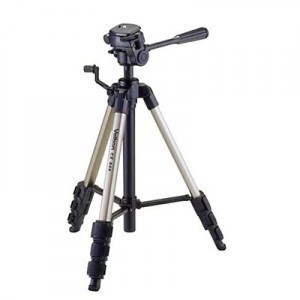•• # Tripod Size Chart

The following sizes and specs are for the Bogen tripods. There are several types of tripods, but these figures can be a helpful gauge of what to expect when you look around.

### Tripod Size Chart: 3001N / 3001BN /3001PRO / 3001BPRO

The 3001N / 3001BN tripods have a load capacity of 11.1 lbs with a thread measuring 3/8" diameter (male thread) with a minimum height of 6.7". The max height is 45.7" (with column). The folded length is 20.9" and the weight is 3.9 lbs. The leg lock type is the locking knob.

The 3001PRO / 3001BPRO have a load capacity of 11.1 lbs and a 3/8" male thread. The minimum height is 8.3"and the max height with column is 46.9". The folded / transport length is 22.1" and the weight is 4.2 lbs. The leg lock type is the flip lever.

### Tripod Size Chart: 190 Series / 3011N / 3011BN

The 190 Series tripods weigh 11.0 lbs and have 3/8" diameter male thread. The minimum height is 16.5" and the max height is 46.8". The leg locks are lever locks and the folded / transport length is 22.6". The tripod weighs 4.9 lbs.

The 3011N / 3011BN tripods have a load capacity of 13.2 lbs and 3/8" diameter male thread. The minimum height is 22.1" and the maximum height is 54.0" with column. The locking mechanism is the locking knob. The folded / transport length is 24.1" and the weight is 4.9 lbs.

### Tripod Size Chart: 055 Series / 3021N / 3021BN

The 055 Series have a load capacity of 13.3 lbs with a 3/8" diameter male thread. The maximum height is 54.3" and the minimum height is 20.0". It uses lever locks and has a folded / transport length of 25.0". The weight is 6.2 lbs.

The 3021N / 3021BN have a load capacity of 13.3 lbs and 3/8" male thread. The minimum height is 4.4" and the maximum height is 53.2". The leg locks are flip levers. The folded / transport length is 25.6" and the weight is 5.3 lbs.

### Tripod Size Chart: 3221GN3 / 3021PRO / 3021BPRO / 3221WN

The 3221GN3 loading capacity is 13.3 lbs and has a 3/8" male thread. The minimum height is 20.0" and the maximum height is 54.3”. It has flip lever leg locks with a folded / transport length of 25.0". The weight is 6.2 lbs.

The 3021PRO / 3021BPRO have a load capacity of 13.3 lbs and have 3/8" male thread. The minimum height is 4.4" and the max height is 53.2". The leg lock type is the flip lever. The folded / transport length is 25.6" and the weight is 5.3 lbs.

The 3221WN load capacity is 13.3 lbs (3/8" in diameter; male thread). The minimum height is 3.2" and 54.6" max. It has flip lever locks. The folded / transport weight is 25.2" and it weighs 6.2 lbs.

This tripod size chart is by no means indicative of the measurements used by other tripod manufacturers. It only serves as a point of reference or comparison.